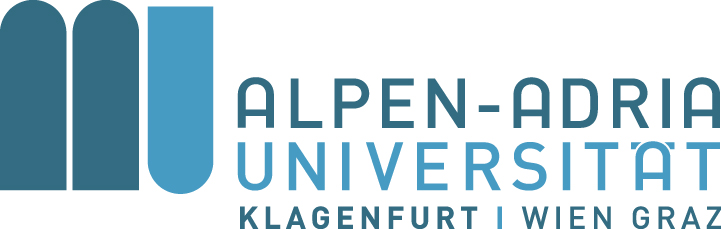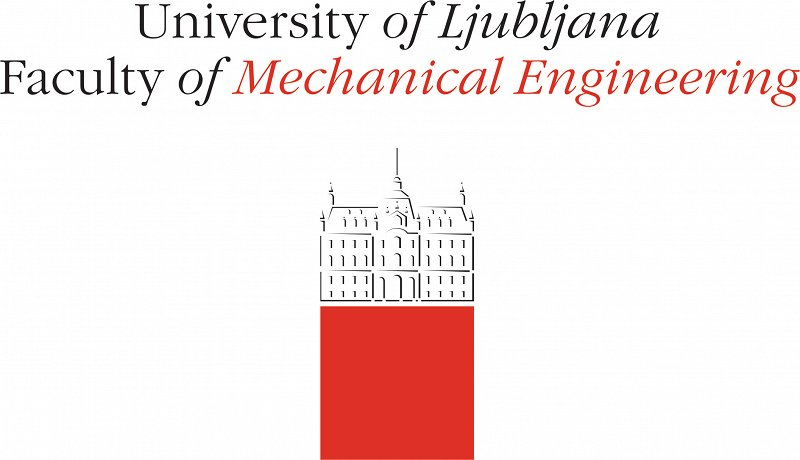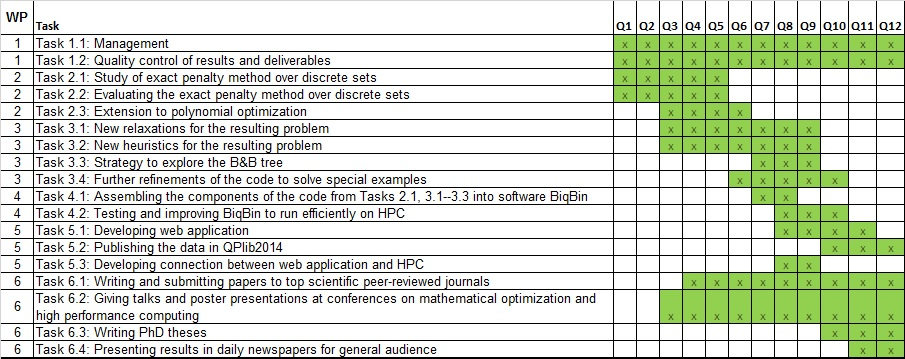Data science is an important driver for many sub-areas in mathematics and computer science. Understanding the data and finding hidden relations between the data instances or variables is getting more and more important, especially in the era of Big Data. Grouping the data instances according to their inner similarty is called clustering analysis [28, Ch.10.3], . When we know the number of groups to be e.g. k, we have the k-clustering problem and when the data instances are the nodes of a graph then this problem is also called a graph k-partitioning problem [5, 10, 45] or community detection problem in network analysis [43, 19].

Suppose matrix D contains the distances between the data instances. Every assignment of n instances into k groups can be represented by a 0/1 matrix X of dimension n  k which has 1 on position (i; j) if and only if the ith instance goes into the jth group. The sum of distances within the groups defined by X is equal to the trace of XTDX. Finding the partition into k parts that minimizes the within-group sum of distances is therefore a non-convex quadratic problem over the set of all binary matrices representing possible partitions. This is an NP-hard problem and data scientists typically solve it approximately using one out of many possible heuristic algorithms [1, 54].

To evaluate the performance of the heuristic algorithms we still need the ground truth, i.e. the optimal solutions of the original clustering problems on small or medium size data sets. Therefore solving (to optimality) the constrained binary quadratic problem on problem instances as big as possible is highly needed. A similar situation can be observed with other examples where binary quadratic problems appear as the mathematical model. Appropriate (meta)heuristic algorithms are used to approximately solve instances of big size and optimal solutions for test instances of small or medium size are needed to evaluate the heuristics.Project activities: